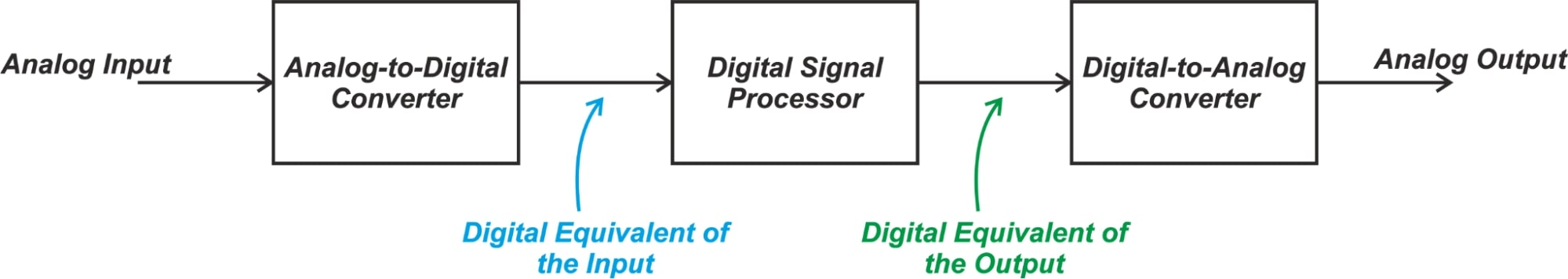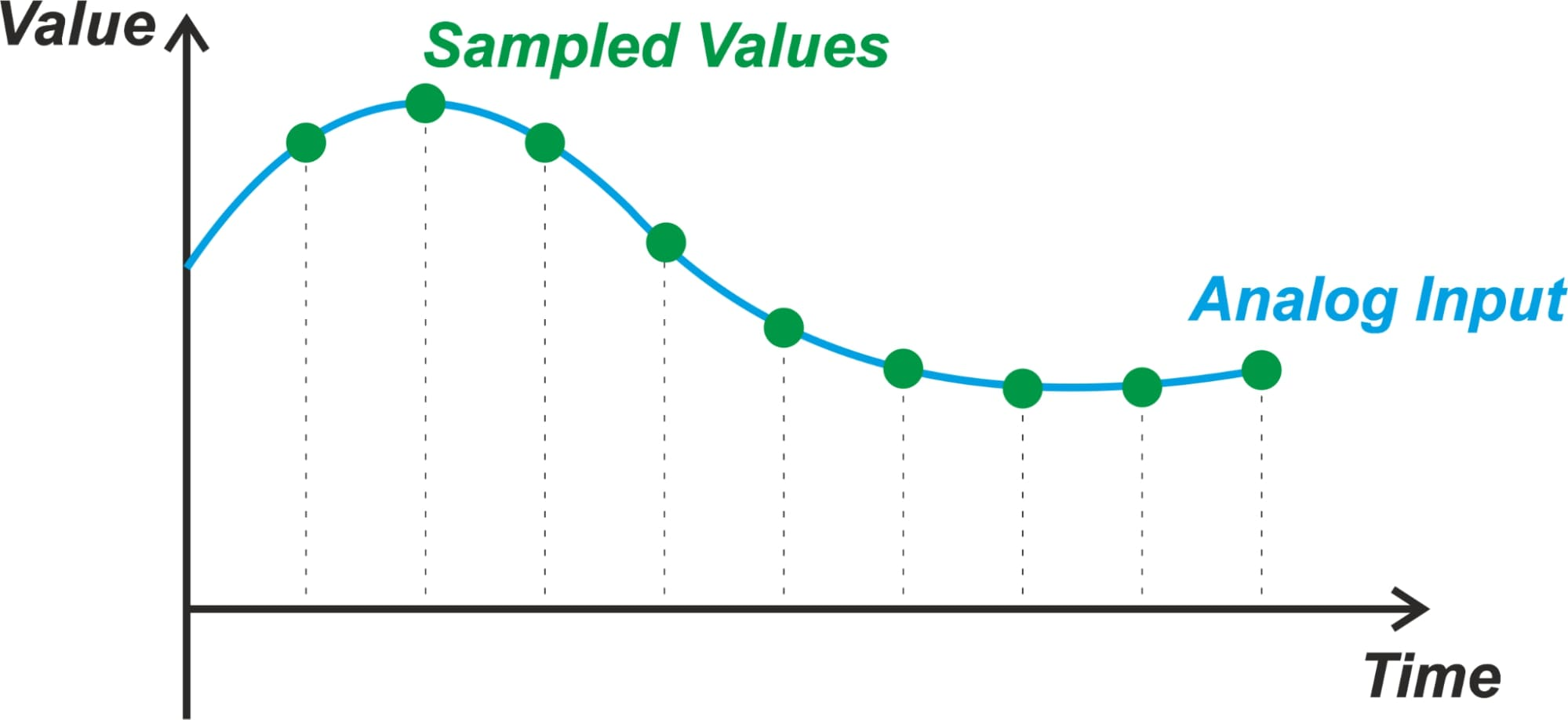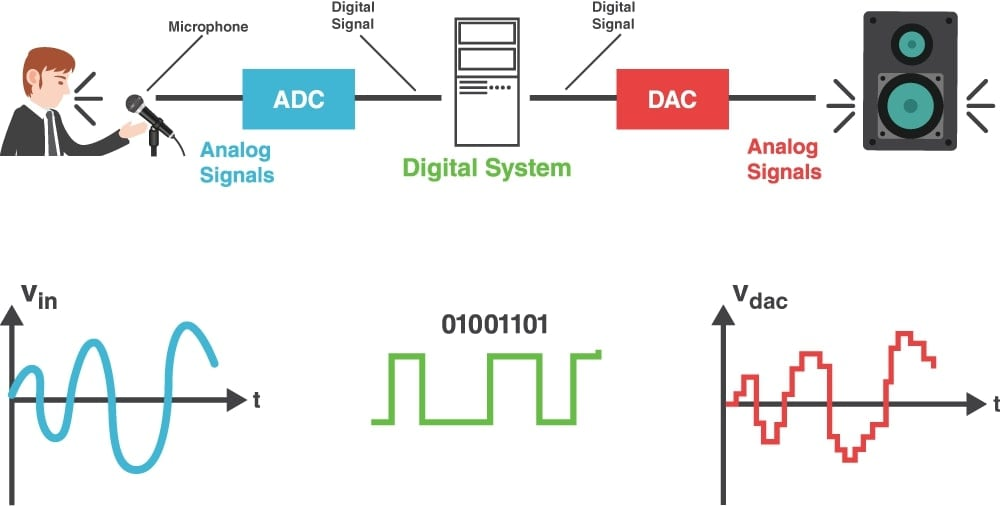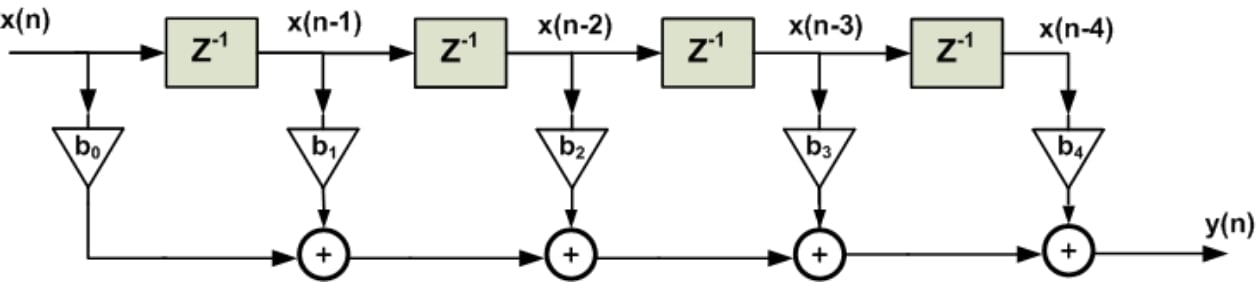Technical Article

# An Introduction to Digital Signal Processing

June 30, 2021 by Dr. Steve Arar

## This article provides a general understanding of what DSP is, how it works, and what advantages it can offer. To appreciate the advantages of DSP, let’s first have a look at the conventional method of signal processing, i.e., analog signal processing.

Digital signal processing (DSP) involves developing algorithms that can be used to enhance a signal in a particular way or extract some useful information from it.

### Analog Signal Processing

Perhaps the simplest analog signal processing example is the familiar RC circuit shown in Figure 1.##### Figure 1.

This circuit acts as a low-pass filter. It removes or filters out the frequency components that are above the circuit cutoff frequency and passes the lower frequency components with little attenuation. In this example, the purpose of signal processing is to eliminate the high-frequency noise and extract the desired part of the signal.

Note that both the input and output are in analog form. This is a big advantage because signals of interest in science and engineering are analog in nature. Hence, with analog signal processing, there is no need for interface circuits (ADCs and DACs) at the input and output of the signal processing block.

### Disadvantages of Analog Signal Processing

One major drawback of analog signal processing is variation in the value of the electrical components. Analog circuits rely on the precision of the active and passive components (resistors, capacitors, inductors, and amplifiers). For example, the cutoff frequency (fC) of the above low-pass filter is given by:

$f_{C} = \frac{1}{2\pi RC}$

As you can see, the filter response is a function of the component values. Since electrical components cannot be manufactured with perfect precision, the accuracy of analog circuits is limited. Due to the component tolerances, the performance is not 100% repeatable and we expect some board-to-board variation in different circuit parameters.

Another disadvantage is that analog circuits are not flexible. For example, to modify the frequency response of the above filter, we need to adjust the value of the components (the hardware needs to be modified). This is not the case with digital signal processing. With DSP, it is even possible to turn a low-pass filter into a high-pass filter by simply changing some programmable coefficients.

Moreover, analog circuits are not suited for implementing mathematical functions (multiplication, division, etc.). This is in contrast to the digital domain where even much more sophisticated mathematical operations can be easily implemented.

### Digital Signal Processing Can Remedy Many Challenges

Digital circuits do not suffer from the above limitations. For example, while variations in the component values and parasitics can slightly change the delay of a CMOS inverter gate, the overall functionality of the gate will be retained. Hence, unlike analog circuits, digital circuits are less susceptible to component variations and parasitics. Digital circuits are also more flexible and suited for implementing mathematical functions.

The remaining question is, what basic components do we need to process the signal in the digital domain.

As shown in Figure 2, we need analog-to-digital (A/D) and digital-to-analog (D/A) converters at the input and output of the signal processing block to interface our digital circuit with the real-world analog signals.### The Role of the A/D converter

The A/D converter samples the analog input at regular intervals as shown in Figure 3.##### Figure 3.

Then, it quantizes the amplitude of each sample. Figure 4 shows how a 4-bit ADC can quantize the analog input.##### Figure 4.

In this figure, the analog input (the blue curve) takes different values in the input range of the ADC. Considering a 4-bit ADC, there are 16 discrete levels to quantize the amplitude of the input signal. These levels are shown by multiples of LSB in the figure. Hence, the LSB (least significant bit) specifies the minimum change in the analog input value that can be detected by the ADC. In other words, it is the minimum change in the input that leads to a change in the ADC output code.

Let’s see how the ADC generates a binary code for each sample. The ADC compares the amplitude of the analog input signal with its 16 discrete levels. Based on this comparison, the digital representation of the input is generated. For example, with the blue curve shown in Figure 4, the process of comparing the input signal with the 16 discrete levels of the ADC may lead to the depicted red curve. Then, the ADC uses a binary code to represent each level of the obtained staircase approximation. For example, when the value of the red curve is equal to 4 times the LSB, the output of our four-bit ADC is 0100.

The point to note is that the “digital signal processor” block in Figure 2 receives a discrete-time sequence because the ADC takes samples at multiples of a pre-specified sampling interval. And, the amplitude of each sample is quantized. This is in contrast to analog signal processing where the input is a continuous-time signal and can take any value in its specified range.

### The Role of the DAC

After the signal is processed by the “Digital Signal Processor” block, we usually need to convert it to an equivalent analog signal. This is achieved by the D/A converter. Figure 5 depicts an audio processing application.##### Figure 5.

In this case, a digital signal processing system is used to add echoes or adjust the tempo and pitch of the voice to get a perfect sound. Then, the processed signal is delivered to the DAC to produce an analog signal that can be outputted by the speakers. Note that there are DSP applications that don't need a DAC. For example, the digital signal processing algorithm employed in a radar might give us the position and speed of an aircraft. This information can simply be printed on paper.

### The “Digital Signal Processor” Block

A DSP algorithm consists of a number of mathematical operations. For example, a 4th order finite impulse response (FIR) filter requires five digital multipliers, four adders along some delay elements as shown below.##### Figure 6.

Hence, a digital signal processor is actually a computing engine. This computing engine can be a general-purpose processor, an FPGA, or even a purpose-built DSP chip. Each option has its own advantages and disadvantages in terms of flexibility, speed, ease of programming, and power consumption.

Since computational resources are very valuable, digital signal processing attempts to provide us with the tools and techniques that enable fast, computationally efficient algorithms. For example, there are several different structures that can be used to implement a given FIR filter.

### DSP Finds Use in a Broad Range of Applications

DSP concepts and tools find use in any application that needs to manipulate the input signal in the digital domain. This includes but is not limited to audio and video compression, speech processing and recognition, digital image processing, and radar applications.

Pursuing a career in each of these areas requires mastering a broad range of specialized DSP algorithms, mathematics, and techniques. In fact, it seems very unlikely for any one individual to master all of the DSP technology that has been already developed. However, some common DSP concepts such as filtering, correlation, and spectral analysis are used in almost all DSP applications. Hence, the first step in DSP education is to master the basic concepts and then focus on the specialized techniques a given field of interest needs.

Some basic concepts of DSP as well as some specialized techniques related to digital image processing are covered in my previous articles. I also have a series on FPGAs and FPGA-based implementation of DSP algorithms that might help you get started with this relatively difficult subject matter.•Rohit khamdekar January 01, 2016
•Jhesus Legarde September 10, 2017